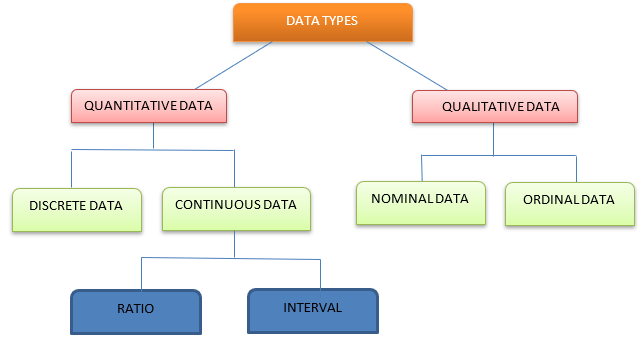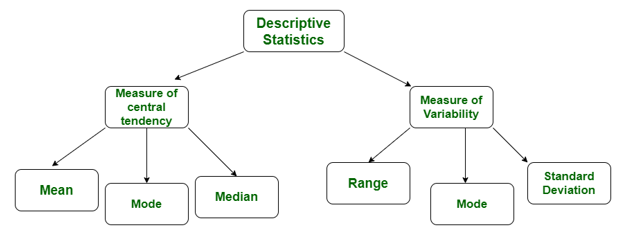﻿ OWSD NIGERIA NATIONAL CHAPTER PRESENTS UNDERSTANDING YOUR DATA TYPE: A KEY TO RIGHT INFERENCE

# OWSD NIGERIA NATIONAL CHAPTER PRESENTS UNDERSTANDING YOUR DATA TYPE: A KEY TO RIGHT INFERENCE

## ABSTRACT

In every scientific research, there is always a degree of uncertainty in the conclusions. This uncertainty is because of some margin of error that has been allowed to cater to some unseen issues that may affect the results. Some of these issues arise through the data, ranging from data sources, data collection, data classification, and data analysis. The ability of a researcher to collect data rightly, understand the type of data and the type of analysis that should be applied to the data, the smaller the uncertainty in the conclusions and more valid the inference. This paper makes a description of data sources, classification of data, and the types of statistical methodology appropriate for each data type, in other for researchers to understand their data and apply the appropriate methodology to arrive at valid results.

KEYWORDS: Data Types, Data Analysis, Statistical methodology, Classification of Data and Data Sources.

## INTRODUCTION:

Scientific research rarely leads to absolute certainty. There is some degree of uncertainty in all conclusions, and statistics allow us to discuss that uncertainty. Statistical methods are used in all areas of science. The Statistical methods explore the difference between (a) proving that something is true and (b) measuring the probability of getting a certain result. It explains how common words like "significant," "control," and "random" have different meanings in the field of statistics than in everyday life.

## What is Data?

Data is a word that describes valid information that can help a researcher answer his/her question(s). It can be understood as the result of observations. There are basically two sources of data which are; primary and secondary sources. Douglas M. (2015) defined a primary source as a source that has not been evaluated or interpreted by anyone else. Data from the primary source are called primary data, they are original and unique data (first-hand data collected by the research), which is directly collected by the researcher from a source such as observations, surveys, questionnaires, case studies, and interviews according to the requirements of the researcher. While data from secondary sources are called secondary data, they are easily accessible but are not pure as they have undergone many statistical treatments and are now in a reformed form. They include government publications, websites, books, journal articles, internal records, etc, Ajayi, V. O. (2017). When working with data it’s crucial to clearly understand some of the main terms, including quantitative (numerical) and qualitative data (categorical) and what their role is. The distinction between categorical and quantitative variables is crucial for deciding which types of data analysis methods to use. The first step towards selecting the right data analysis method today is to understand the type of data available to you and the purpose of the study/experiment.

## DEFINITIONS:

• Categorical data or Qualitative data consist of categorical values or variables, where the data are represented in labeled or given a name. Such as the breed of a dog, the color of the car, gender, religious affiliation, and so on.
• Numerical data or Quantitative data comprise numbers or numerical values that represent the data, such as height, weight, age of a person, etc.Figure 1: Types of data representation

### CATEGORICAL DATA:

There are two types of categorical data, namely; the nominal and ordinal data.

• Nominal Data

This is a type of data used to name variables without providing any numerical value. Coined from the Latin nomenclature “Nomen” (meaning name), this type of data does not have any form of ordering.  Examples of nominal data include name, religious affiliation, hair colour, sex, etc. It is mostly collected using surveys or questionnaires; this data type is descriptive.

• Ordinal Data

This is a data type with a set order or scale to it. However, this order does not have a standard scale on which the difference in variables in each scale is measured. Although mostly classified as categorical data, it is can exhibit both categorical and numerical data characteristics sometimes. Its classification under ordinal categorical data has to do with the fact that it has an order to it. With this scale, we can determine the direction of the difference of a variable, but we cannot determine the size of the difference. Some ordinal data examples include; the Likert scale, interval scale, disease severity, educational level, etc. Each of these examples may have different collection and analysis techniques, but they are all ordinal data.

### QUANTITATIVE DATA:

There are two types of quantitative data:—discrete and continuous:Figure 1: Discrete data        Figure 2: Continuous data

• ## Discrete data

Discrete data is a type of numerical data with countable elements. This type of data includes incidences, proportions, or characteristics that are counted in non-negative integers. One example of this is the number of cars parked in Ofrima car park.

• ## Continuous data

Continuous data is a numerical data type with uncountable elements. It can be any value (no matter how big or small) measured on a limitless scale. For example, the measure of time, temperature, etc.

Continuous data can be further classified into interval data or ratio data:

### INTERVAL DATA

Interval data can be measured along a continuum, where there is an equal distance between each point on the scale. It specifies that the distances between each interval on the scale are equivalent along the scale from low interval to high interval. Interval data has no true or meaningful zero value. For instance, the difference between 5 and 6 feet is equal to the difference between 25 and 50 miles on a scale.

### RATIO DATA

Ratio data is similar to interval data in that it’s equally spaced on a scale, but unlike interval data, ratio data has a true zero. Weight is classified as ratio data; whether it has equal weight or weighs zero grams—it weighs nothing at all.

## METHODS OF DATA ANALYSIS

The two main branches of statistical analysis are; descriptive and inferential statistics. Descriptive statistics describe your sample data that is it helps you make sense of your sample data whereas inferential statistics make predictions about what you’ll find in the population. Descriptive statistics focuses on describing the sample, while inferential statistics aim to make predictions about the population, based on the findings within the sample (Population is the entire group of people/entities you’re interested in, and the sample is the subset of the population that you can actually get access to). Descriptive statistics helps to inform you of which inferential statistical techniques you can use; it helps you spot potential errors in the data and also helps you understand both the big picture and the finer details. Common descriptive statistical methods include mean, median, standard deviation, skewness, etc.Inferential statistics allow you to connect the dots and make predictions about what you expect to see in the real world population, based on what you observe in your sample data. It makes predictions about differences between groups and also predicts relationships between variables; some inferential statistical methods include t-tests, ANOVA, correlation, regression analysis, etc.

To choose the right statistical methods and techniques, you need to consider the type of data you are working with, as well as your research questions and hypotheses. Importantly every statistical method has its own assumptions and limitations.

## Quantitative data analysis:

This is all about analyzing number-based data using various statistical techniques. Two popular quantitative data analysis techniques: regression analysis and hypothesis analysis.

• Regression analysis is a type of statistical analysis method that determines the relationships between independent and dependent variables. In finance, regression is defined as a method to help investment and financial managers value assets and determine variable relationships in commodity prices and stocks.
• Hypothesis analysis is a data analysis technique that uses sample data to test a hypothesis. Hypothesis analysis is a statistical test method to validate an assumption and determine if it’s plausible or factual. In this approach, an analyst develops two hypotheses — only one of them can be true. Two foundational components of hypothesis analysis are the null hypothesis and the alternative hypothesis.

Qualitative data analysis: Qualitative data analysis techniques are built on two main qualitative data approaches: deductive and inductive.

• Deductive approach. This analysis method is used by researchers and analysts who already have a theory or a predetermined idea of the likely input from a sample population. The deductive approach aims to collect data that can methodically and accurately support a theory or hypothesis.• Inductive approach. In this approach, a researcher or analyst with little insight into the outcome of a sample population collects the appropriate and proper amount of data about a topic of interest. Then, they investigate the data to look for patterns. The aim is to develop a theory to explain patterns found in the data.## REFERENCES:

Ajayi, V. O. (2017). Primary Sources of Data and Secondary Sources of Data.  https://www.researchgate.net/publication/320010397. DOI: 10.13140/RG.2.2.24292.68481

Derek Jansen and Kerryn Warren (2020). Quantitative Data Analysis 101, https://gradcoach.com/quantitative-data-analysis-methods/

Douglas, M. (2015). “Sources of data”. Retrieved on 22nd September, 2017 from http://www.onlineetymologydictionary/data

https://www.cuemath.com/data/discrete-data/, Discrete Data.

https://www.formpl.us/blog/categorical-data, Categorical Data: Definition + [Examples, Variables & Analysis]